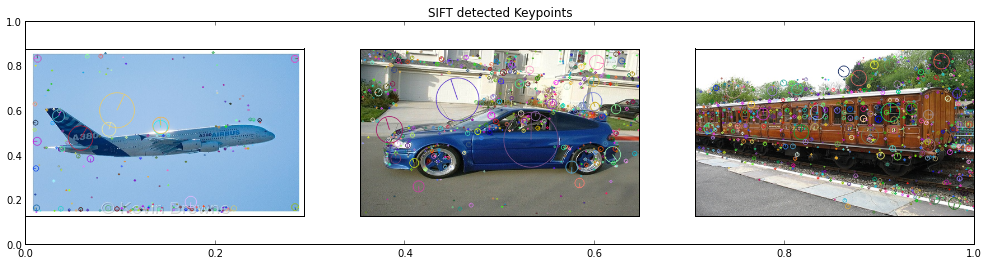# Visual Categorization with Bags of Keypoints in Shogun¶

#### By Abhijeet Kislay (GitHub ID: kislayabhi)¶

This notebook is about performing Object Categorization using SIFT descriptors of keypoints as features, and SVMs to predict the category of the object present in the image. Shogun's K-Means clustering is employed for generating the bag of keypoints and its k-nearest neighbours module is extensively used to construct the feature vectors.

## Background¶

This notebook presents a bag of keypoints approach to visual categorization. A bag of keypoints corresponds to a histogram of the number of occurences of particular image patterns in a given image.The main advantages of the method are its simplicity, its computational efficiency and its invariance to affine transformations, as well as occlusion, lighting and intra-class variations.

## Strategy¶

1. Compute (SIFT) descriptors at keypoints in all the template images and pool all of them together

### SIFT¶

SIFT extracts keypoints and computes its descriptors. It requires the following steps to be done:

• Scale-space Extrema Detection: Difference of Gaussian (DOG) are used to search for local extrema over scale and space.
• Keypoint Localization: Once potential keypoints are found, we refine them by eliminating low-contrast keypoints and edge keypoints.
• Orientation Assignment: Now an orientation is assigned to each keypoint to achieve invariance to image rotation.
• Keypoint Descriptor: Now a keypoint descriptor is created. A total of 128 elements are available for each keypoint.

To get more details about SIFT in OpenCV, do read OpenCV python documentation here.

OpenCV has a nice API for using SIFT. Let's see what we are looking at:

In :
#import Opencv library
try:
import cv2
except ImportError:
print "You must have OpenCV installed"
exit(1)

#check the OpenCV version
try:
v=cv2.__version__
assert (tuple(map(int,v.split(".")))>(2,4,2))
except (AssertionError, ValueError):
print "Install newer version of OpenCV than 2.4.2, i.e from 2.4.3"
exit(1)

import numpy as np
import matplotlib.pyplot as plt
%matplotlib inline
from modshogun import *

# get the list of all jpg images from the path provided
import os
def get_imlist(path):
return [[os.path.join(path,f) for f in os.listdir(path) if (f.endswith('.jpg') or f.endswith('.png'))]]

#Use the following function when reading an image through OpenCV and displaying through plt.
def showfig(image, ucmap):
#There is a difference in pixel ordering in OpenCV and Matplotlib.
#OpenCV follows BGR order, while matplotlib follows RGB order.
if len(image.shape)==3 :
b,g,r = cv2.split(image)       # get b,g,r
image = cv2.merge([r,g,b])     # switch it to rgb
imgplot=plt.imshow(image, ucmap)
imgplot.axes.get_xaxis().set_visible(False)
imgplot.axes.get_yaxis().set_visible(False)


We try to construct the vocabulary from a set of template images. It is a set of three general images belonging to the category of car, plane and train.

OpenCV also provides cv2.drawKeyPoints() function which draws the small circles on the locations of keypoints. If you pass a flag, cv2.DRAW_MATCHES_FLAGS_DRAW_RICH_KEYPOINTS to it, it will draw a circle with size of keypoint and it will even show its orientation. See below example.

In :
plt.rcParams['figure.figsize'] = 17, 4
filenames=get_imlist('../../../data/SIFT/template/')
filenames=np.array(filenames)

# for keeping all the descriptors from the template images
descriptor_mat=[]

# initialise OpenCV's SIFT
sift=cv2.SIFT()
fig = plt.figure()
plt.title('SIFT detected Keypoints')

for image_no in xrange(3):
img=cv2.imread(filenames[image_no])
img=cv2.resize(img, (500, 300), interpolation=cv2.INTER_AREA)
gray=cv2.cvtColor(img, cv2.COLOR_BGR2GRAY)
gray=cv2.equalizeHist(gray)

#detect the SIFT keypoints and the descriptors.
kp, des=sift.detectAndCompute(gray,None)
# store the descriptors.
descriptor_mat.append(des)
# here we draw the keypoints
img=cv2.drawKeypoints(img, kp, flags=cv2.DRAW_MATCHES_FLAGS_DRAW_RICH_KEYPOINTS)
fig.add_subplot(1, 3, image_no+1)
showfig(img, None)2. Group similar descriptors into an arbitrary number of clusters.

We take all the descriptors that we got from the three images above and find similarity in between them. Here, similarity is decided by Euclidean distance between the 128-element SIFT descriptors. Similar descriptors are clustered into k number of groups. This can be done using Shogun's **KMeans class**. These clusters are called bags of keypoints or visual words and they collectively represent the vocabulary of the program. Each cluster has a cluster center, which can be thought of as the representative descriptor of all the descriptors belonging to that cluster. These cluster centers can be found using the **get_cluster_centers()** method.

To perform clustering into k groups, we define the get_similar_descriptors() function below.

In :
def get_similar_descriptors(k, descriptor_mat):

descriptor_mat=np.double(np.vstack(descriptor_mat))
descriptor_mat=descriptor_mat.T

#initialize KMeans in Shogun
sg_descriptor_mat_features=RealFeatures(descriptor_mat)

#EuclideanDistance is used for the distance measurement.
distance=EuclideanDistance(sg_descriptor_mat_features, sg_descriptor_mat_features)

#group the descriptors into k clusters.
kmeans=KMeans(k, distance)
kmeans.train()

#get the cluster centers.
cluster_centers=(kmeans.get_cluster_centers())

return cluster_centers

In :
cluster_centers=get_similar_descriptors(100, descriptor_mat)


3. Now, compute training data for the SVM classifiers. .

Since we have already constructed the vocabulary, our next step is to generate viable feature vectors which can be used to represent each training image so that we can use them for multiclass classification later in the code.

• We begin by computing SIFT descriptors for each training image.
• For each training image, associate each of its descriptors with one of the clusters in the vocabulary. The simplest way to do this is by using k-Nearest Neighbour approach. This can be done using Shogun's KNN class. Euclidean distance measure is used here for finding out the neighbours.
• Making a histogram from this association. This histogram has as many bins as there are clusters in the vocabulary. Each bin counts how many descriptors in the training image are associated with the cluster corresponding to that bin. Intuitively, this histogram describes the image in the visual words of the vocabulary, and is called the bag of visual words descriptor of the image.

In short, we approximated each training image into a k element vector. This can be utilized to train any multiclass classifier.

First, let us see a few training images

In :
# name of all the folders together
folders=['cars','planes','trains']
training_sample=[]
for folder in folders:
#get all the training images from a particular class
filenames=get_imlist('../../../data/SIFT/%s'%folder)
for i in xrange(10):
temp=cv2.imread(filenames[i])
training_sample.append(temp)

plt.rcParams['figure.figsize']=21,16
fig=plt.figure()
plt.title('10 training images for each class')
for image_no in xrange(30):
fig.add_subplot(6,5, image_no+1)
showfig(training_sample[image_no], None)